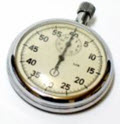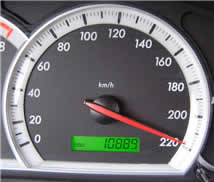# "Unit" of Measurement

In Measurement we talk about "Units" ... what are they?

A unit is any measurement that there is 1 of.

So 1 meter is a unit.

And 1 second is also a unit

And 1 m/s (one meter per second) is also a unit, because there is one of it.

And so on ...

## Without the "1"

It is also common to drop the "1" in front and just talk about the type of measurement as a unit.Example: a commonly used unit of time is the second

We don't say a stopwatch measures "1 seconds", we say it measures "seconds".

So "Unit" is a general term that means the type of measurement. And people understand that we mean just "1" of it.

So a conversation might go like this

Alex: "It measures 100"
Sam: "In what Unit?"
Alex: "Centimeters"

## Example: SpeedometerWhat unit does this Speedometer measure? It measures km/h (kilometers per hour) So "220" means 220 kilometers per hour.

## Abbreviation

We usually write units just using their abbrevations.

### Example: m/s (or m s-1) for meter per second

m/s is a unit of speed

### Example: kg/m3 (or kg m-3) for kilogram per cubic meter

kg/m3 is a unit of density: how much mass per unit of volume.

## Standardized

Units of Measurement are "standardized", meaning that there is a well-defined standard way to measure 1 of them.### Example: For many years (1889 to 1960) there was the International Prototype Metre bar to show people exactly what 1 meter was.

But that wasn't accurate enough!

Now "1 Meter" is defined as how far light travels in 1/299,792,458 of a second.

## Different Systems of Measurement

Units can be grouped together to make a "System".

Example: the meter, kilogram and second (together with a few other units) together make up the "SI" Metric System of Measurement.

Example: the inch, foot, yard and mile are the units of length in the US Standard System of Measurement

## Unit Price

Unit Price tells us the cost per liter, per kilogram, per pound, etc, of what we want to buy.

It is a good way of comparing costs of what we buy.

Example: What is best

• 2 liters of Milk at \$3.80, or
• 1.5 liters of Milk at \$2.70 ?

In this case the "Unit" is 1 liter, and the Unit Prices are:

• \$3.80 / 2 liters = \$1.90 per liter
• \$2.70 / 1.5 liters = \$1.80 per liter

So the lowest Unit Price (and the best bargain) is 1.5 liter at \$2.70.